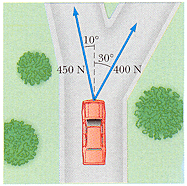# Problem: Two forces are applied to a car in an effort to move it, as shown in Figure P4.12.(a) What is the resultant of these two forces?magnitudedirection(b) If the car has a mass of 3100 kg, what magnitude acceleration does it have? Ignore friction.

###### FREE Expert Solution

In this problem, we're going to use:

The equation for vector magnitude given by,

$\overline{)\mathbf{|}\stackrel{\mathbf{⇀}}{\mathbit{A}}\mathbf{|}{\mathbf{=}}\sqrt{{{\mathbit{A}}_{\mathbit{x}}}^{\mathbf{2}}\mathbf{+}{{\mathbit{A}}_{\mathbit{y}}}^{\mathbf{2}}}}$

Direction:

$\overline{){\mathbf{tan}}{\mathbit{\theta }}{\mathbf{=}}\frac{{\mathbit{A}}_{\mathbit{y}}}{{\mathbit{A}}_{\mathbit{x}}}}$

91% (413 ratings)###### Problem Details

Two forces are applied to a car in an effort to move it, as shown in Figure P4.12.(a) What is the resultant of these two forces?
magnitude
direction
(b) If the car has a mass of 3100 kg, what magnitude acceleration does it have? Ignore friction.